# McGraw Hill Math Grade 5 Chapter 10 Lesson 2 Answer Key Identifying and Measuring Angles

All the solutions provided in McGraw Hill Math Grade 5 Answer Key PDF Chapter 10 Lesson 2 Identifying and Measuring Angles are as per the latest syllabus guidelines.

## McGraw-Hill Math Grade 5 Answer Key Chapter 10 Lesson 2 Identifying and Measuring Angles

Identify and Measure

Classify each angle as straight, right, obtuse, or acute. Measure each angle with a protractor. You may have to extend the rays of an angle to measure it. Write each measure?

Question 1.Question 2.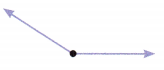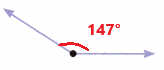Obtuse angle
Explanation:
In the above image we can observe the measured angle 147 degrees. The angle which measures greater than 90 degrees and less than 180 degrees is called as obtuse angle. So, the above angle is obtuse angle.

Question 3.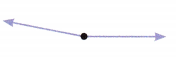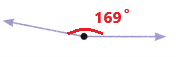Obtuse angle
Explanation:
In the above image we can observe the measured angle 169 degrees. The angle which measures greater than 90 degrees and less than 180 degrees is called as obtuse angle. So, the above angle is obtuse angle.

Question 4.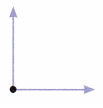Right angle
Explanation:
In the above image we can observe the measured angle 90 degrees. The angle which measures exactly 90 degrees is called as right angle. So, the above angle is right angle.

Question 5.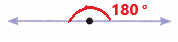Straight angle
Explanation:
In the above image we can observe the measured angle 180 degrees. The angle which measures 180 degrees is called as straight angle. So, the above angle is straight angle.

Question 6.